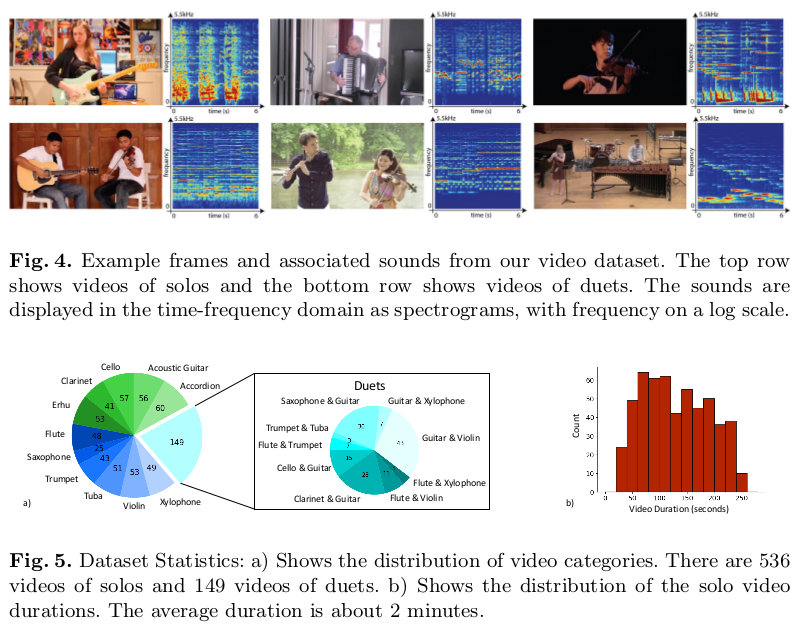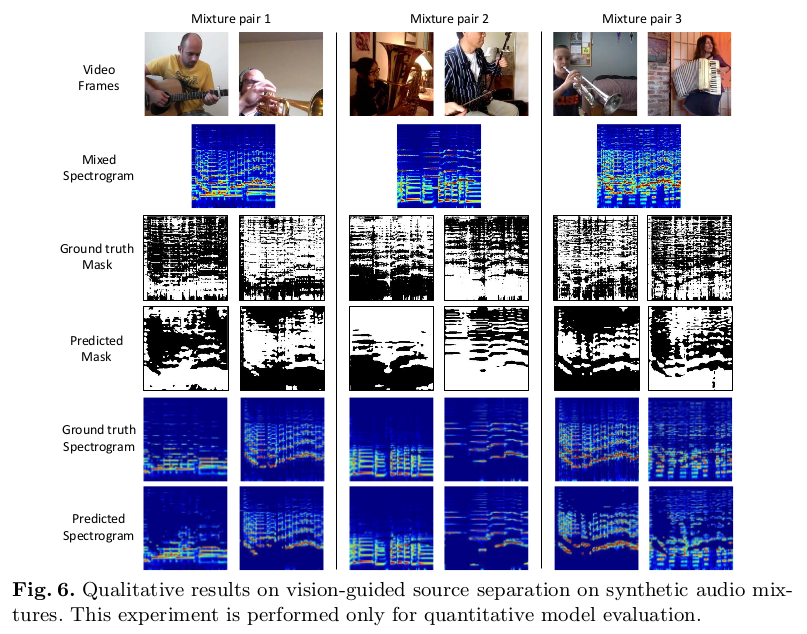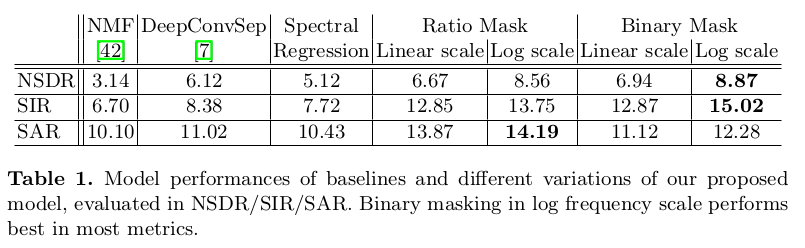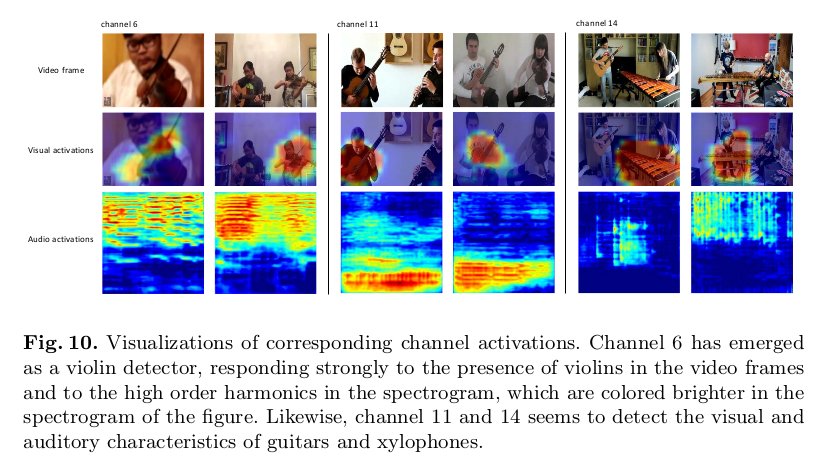# 论文引入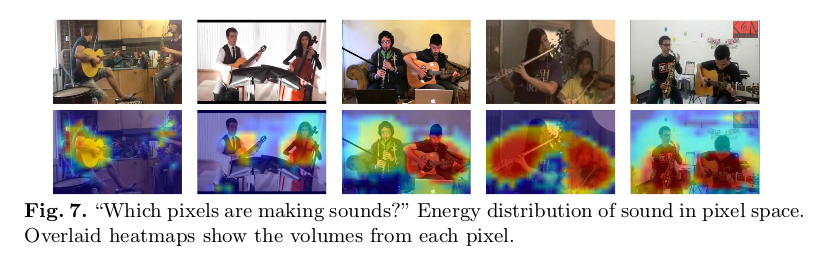• 利用视觉信号引导混合声音的分离
• 视觉信号辅助下可以实现声音的定位
• 视听觉关联性优化网络，实现自监督学习

# PixelPlayer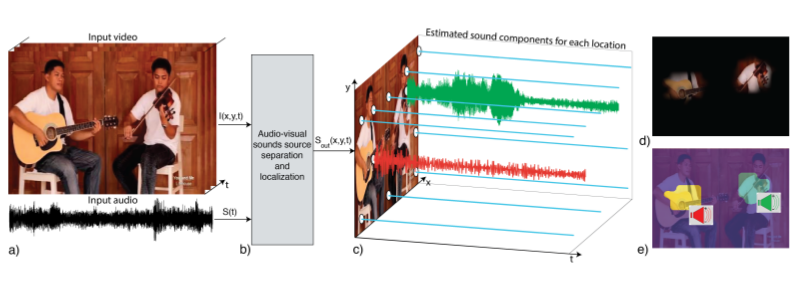# 模型框架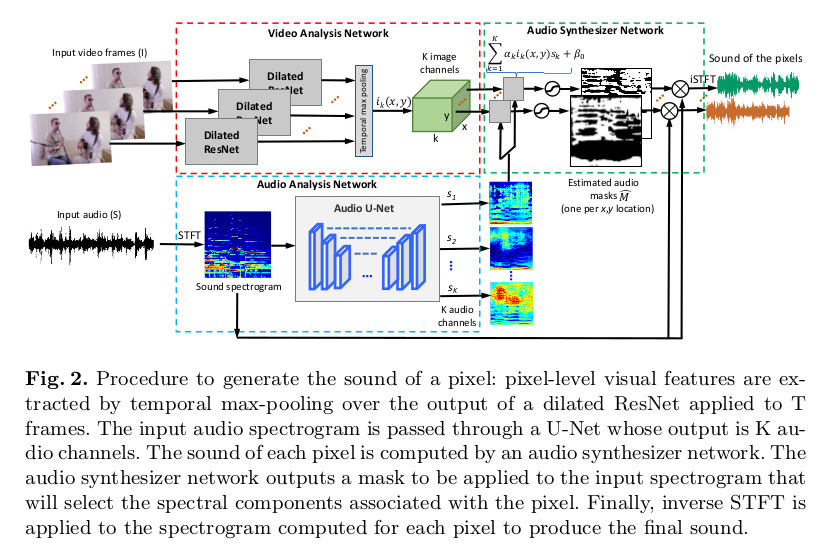# Audio U-Net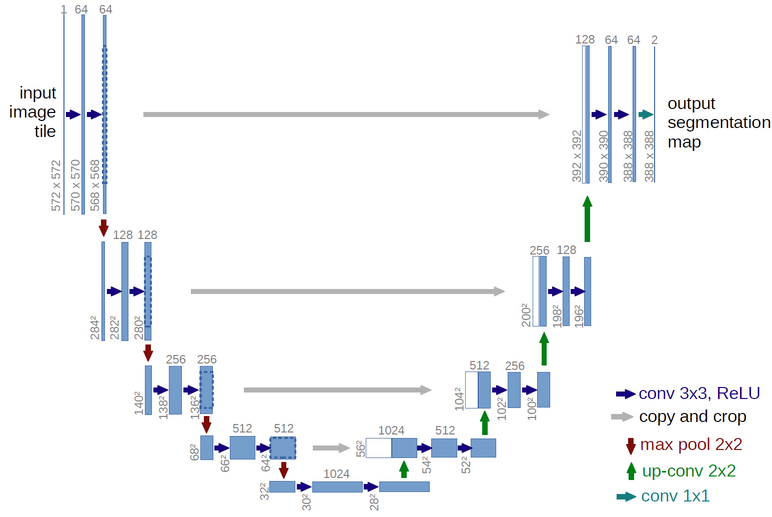# 模型训练

$\begin{equation} M_n(u,v) = [[S_n(u,v) \geq S_m(u,v)]],\forall m=(1,...,N) \end{equation}$

$\begin{equation} M_n(u,v) = \frac{S_n(u,v)}{S_{mix}(u,v)} \end{equation}$

# 实验